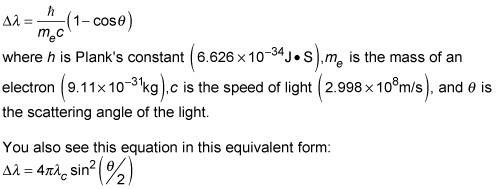##### Quantum Physics Workbook For Dummies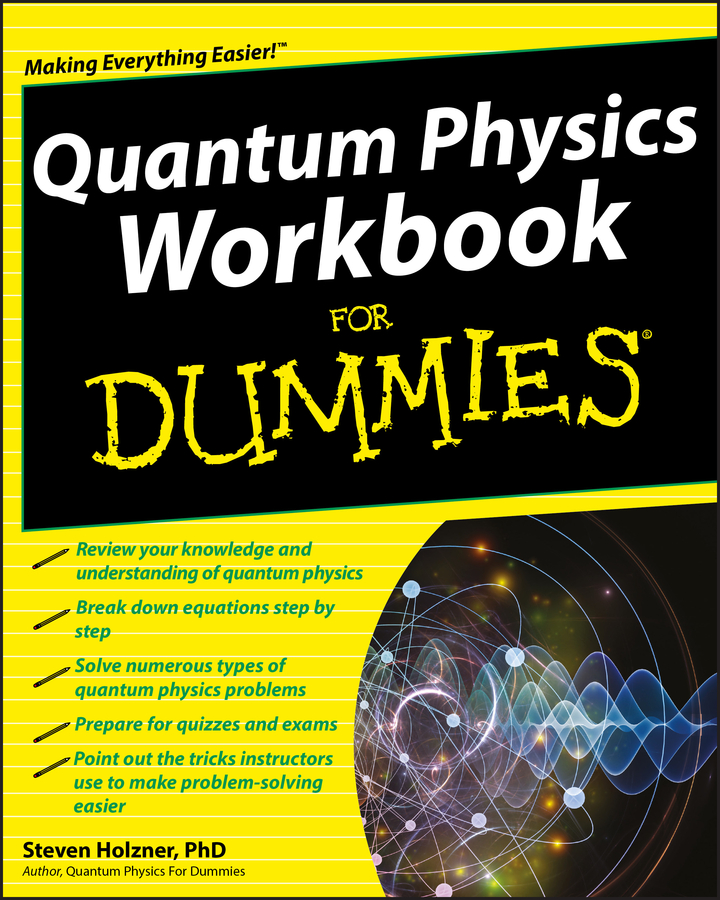In dabbling in quantum physics, you come across spin operators, commutation relationships, and many formulae and principles. You also learn about various effects named for people, such as the Hamiltonian, the Heisenberg Uncertainty Principle, the Schrödinger Equation, and the Compton Effect.

This Cheat Sheet provides a quick reference to some of the main equations used in quantum physics.

## Quantum physics and the Hamiltonian

One of the central problems of quantum mechanics is to calculate the energy levels of a system. The energy operator, called the Hamiltonian, abbreviated H, gives you the total energy. Finding the energy levels of a system breaks down to finding the eigenvalues of the problem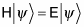The eigenvalues can be found by solving the equation: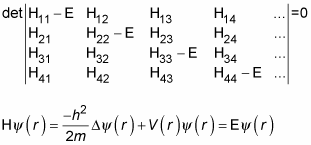## The Heisenberg uncertainty principle

In quantum physics, you encounter the Heisenberg uncertainty principle, which says that the better you know the position of a particle, the less you know the momentum, and vice versa. In the x direction, for example, that looks like this: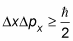where Dx is the measurement uncertainty in the particle’s x position, Dpx is its measurement uncertainty in its momentum in the x direction, and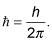This relation holds for all three dimensions: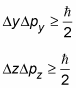## The Schrödinger equation

When a quantum mechanical state can be described by a wave function,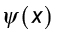then this is a solution of the Schrödinger equation, which is written in terms of the potential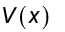and energy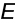like so: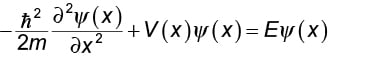The Schrödinger equation work in three dimensions as well: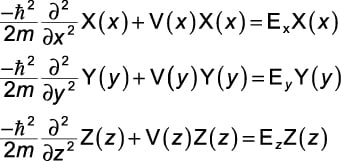## Spin operators and commutation in quantum physics

Don’t think quantum physics is devoid of anything but dry science. The fact is that it’s full of relationships, they’re just commutation relationships — which are pretty dry science after all. In any case, among the angular momentum operators Lx, Ly, and Lz, are these commutation relations: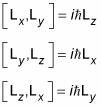All the orbital angular momentum operators, such as Lx, Ly, and Lz, have analogous spin operators: Sx, Sy, and Sz. And the commutation relations work the same way for spin: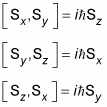## The Compton effect

In quantum physics, you may deal with the Compton effect of X-ray and gamma ray qualities in matter. To calculate these effects, use the following formula, which assumes that the light is represented by a photon with energy E = hu and that its momentum is p = E/c: